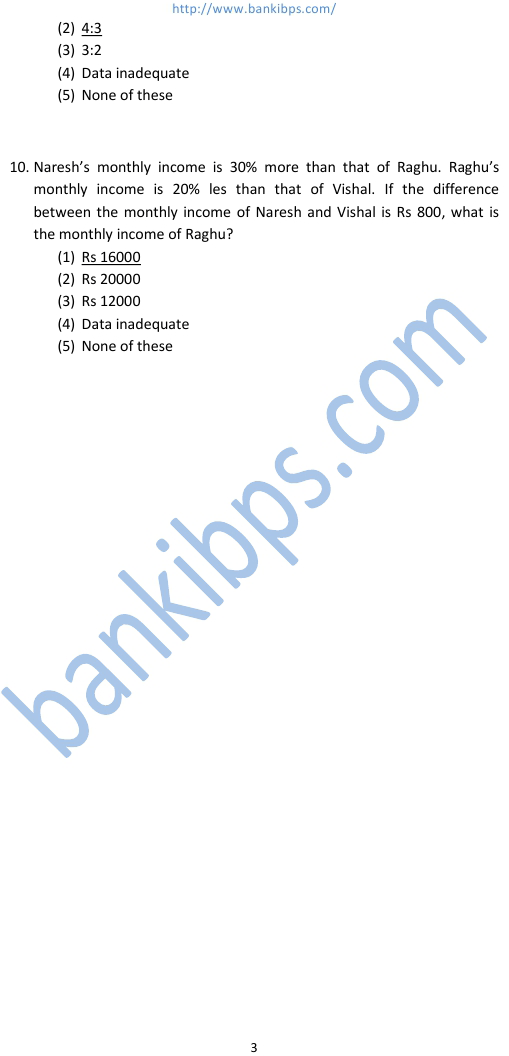# Bank Exam Aptitude Questions

What approximate value should come in place of the question mark (?) in the following equation? 152v(?) + 795 = 8226 – 3486 425 985 1225 1025 675 bank exam aptitude questions Two fifth of one forth of three sevenths of a number is 15. What is half of that number? 96 196 94 188 None of these Ashok borrowed some money at the rate of 6 p.c.p.a for the first two years, at the rate of 9 pcpa for the next three years and at the rate of 14 pcpa for the period beyond five years. If he pays a total interest of Rs 11400 at the end of nine years, how much money did he borrow? Rs 16000 Rs 14000 Rs 18000 Rs 12000 None of these Twenty-four men can complete a work in sixteen days. Thirty-two women can complete the same work in twenty-four days. Sixteen men and sixteen women started working and worked for twelve days. How many more men are to be added to complete the remaining work in 2 days? 48 24 36 16 None of the above
Practice Exercise - 337 [ Aptitude ]